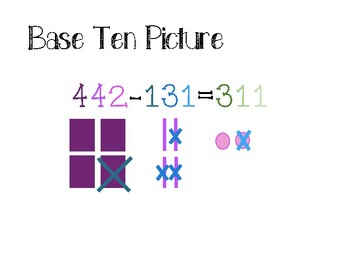# Subtraction Strategies Anchor ChartsSubject
Resource Type
File Type

PDF

(7 MB)
Product Rating
Standards
• Product Description
• StandardsNEW

Hang these strategies in your room for easy access for all students to see. Your students will feel confident when it comes to subtracting multi digit problems.

Need help solving multiplication and division problems too? Check out these anchor charts for all your math needs!

Multiplication Two-digit by Two-digit Anchor Charts

Division Anchor Charts

Multiplication and Division Anchor Charts

Use addition and subtraction within 100 to solve one- and two-step word problems involving situations of adding to, taking from, putting together, taking apart, and comparing, with unknowns in all positions, e.g., by using drawings and equations with a symbol for the unknown number to represent the problem.
Fluently add and subtract within 1000 using strategies and algorithms based on place value, properties of operations, and/or the relationship between addition and subtraction.
Use place value understanding to round whole numbers to the nearest 10 or 100.
Explain why addition and subtraction strategies work, using place value and the properties of operations.
Add and subtract within 1000, using concrete models or drawings and strategies based on place value, properties of operations, and/or the relationship between addition and subtraction; relate the strategy to a written method. Understand that in adding or subtracting three-digit numbers, one adds or subtracts hundreds and hundreds, tens and tens, ones and ones; and sometimes it is necessary to compose or decompose tens or hundreds.
Total Pages
N/A
N/A
Teaching Duration
N/A
Report this Resource to TpT
Reported resources will be reviewed by our team. Report this resource to let us know if this resource violates TpT’s content guidelines.